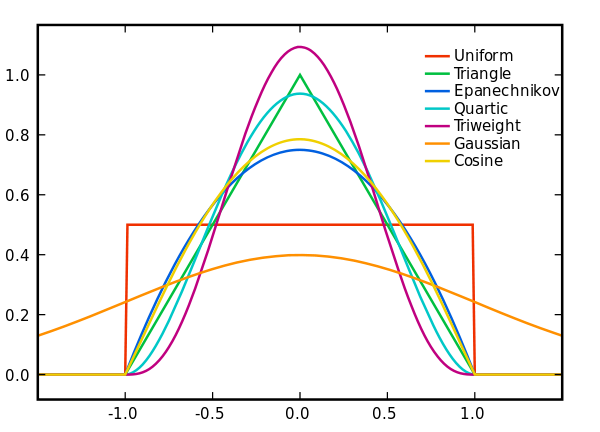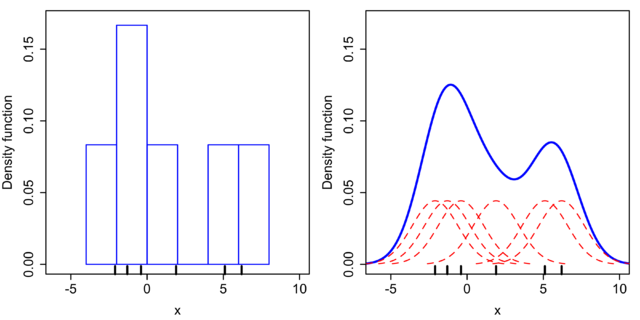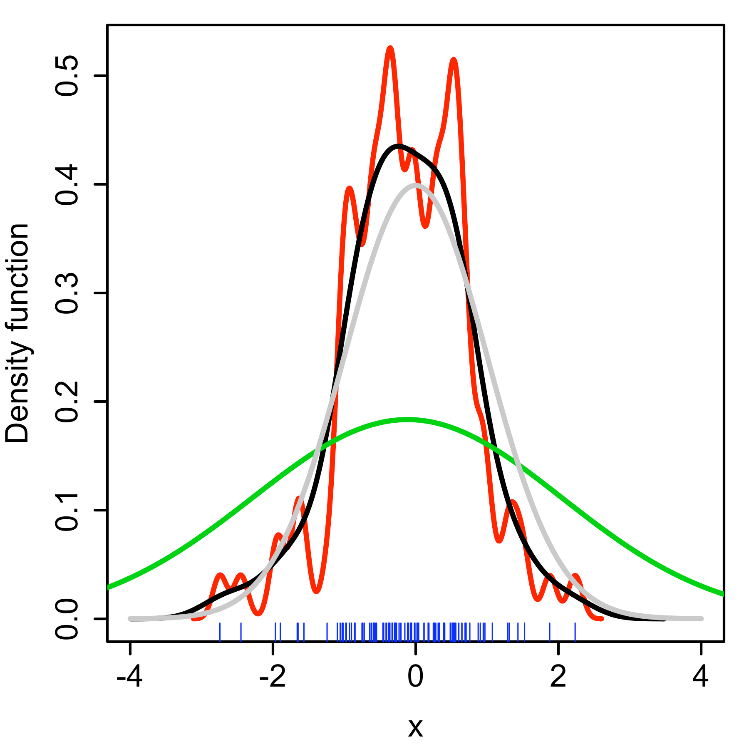# 核密度估计与自适应带宽的核密度估计

（若有闲暇，后续将极大似然、贝叶斯估计等等估计方法详细总结）

f^h(x)=1ni=1nKh(xxi)=1nhi=1nK(xxih)x1=2.1x2=1.3x3=0.4x4=1.9x5=5.1x6=6.2 $x_1 = -2.1，x_2 = -1.3，x_3 = -0.4，x_4 = 1.9，x_5 = 5.1，x_6 = 6.2$MISE(h)=E[(f^h(x)f(x))2dx]

weakassumptions $weak\, assumptions$下， MISE(h)=AMISE(h)+o(1nh+h4) $MISE (h) =AMISE(h) + o(\frac{1}{nh} + h^4)$，其中 AMISE $AMISE$为渐进的 MIS $MIS$E。而 AMISE $AMISE$有，

AMISE(h)=R(K)nh+14m2(K)2h4R(f′′)

R(g)=g(x)2dx

m2(K)=x2K(x)dx

AMISE(h)h=R(K)nh2+m2(K)2h3R(f′′)=0

hAMISE=R(K)15m2(K)25R(f′′)15n15

h=(4σ^53n)151.06σ^n15

1.在数据可视化的相关领域中，带宽的大小决定了核密度估计函数（KDE）的平滑（smooth）程度，带宽越小越undersmooth，带宽越大越oversmooth。（详细解释）
2.在POI兴趣点推荐领域，或位置服务领域，带宽 h $h$的设置主要与分析尺度以及地理现象特点有关。较小的带宽可以使密度分布结果中出现较多的高值或低值区域，适合于揭示密度分布的局部特征，而较大的带宽可以在全局尺度下使热点区域体现得更加明显。另外，带宽应与兴趣点的离散程度呈正相关，对于稀疏型的兴趣点分布应采用较大的带宽，而对于密集型的兴趣点则应考虑较小一些的带宽。

k(x)=1Mj1M1(ωhj)nK(xx(j)ωhj)

K(x)=1(2π)n|S|exp(12xTS1x)

hj=[Mk=1f(x(k))]1Mf(x(j))α

https://en.wikipedia.org/wiki/Kernel_density_estimation
https://en.wikipedia.org/wiki/Variable_kernel_density_estimation
http://www.tuicool.com/articles/EVJnI3

02-22453
11-1614万+

12-1511万+
05-26
04-242818
09-253153
10-101271
07-131万+
01-141346
03-19340
02-054745
03-289159
11-051万+
03-155万+
06-198142
11-209832
12-149万+

### “相关推荐”对你有帮助么？

•非常没帮助
•没帮助
•一般
•有帮助
•非常有帮助¥2 ¥4 ¥6 ¥10 ¥20余额支付 (余额：-- )扫码支付获取中扫码支付点击重新获取扫码支付1.余额是钱包充值的虚拟货币，按照1:1的比例进行支付金额的抵扣。
2.余额无法直接购买下载，可以购买VIP、C币套餐、付费专栏及课程。余额充值